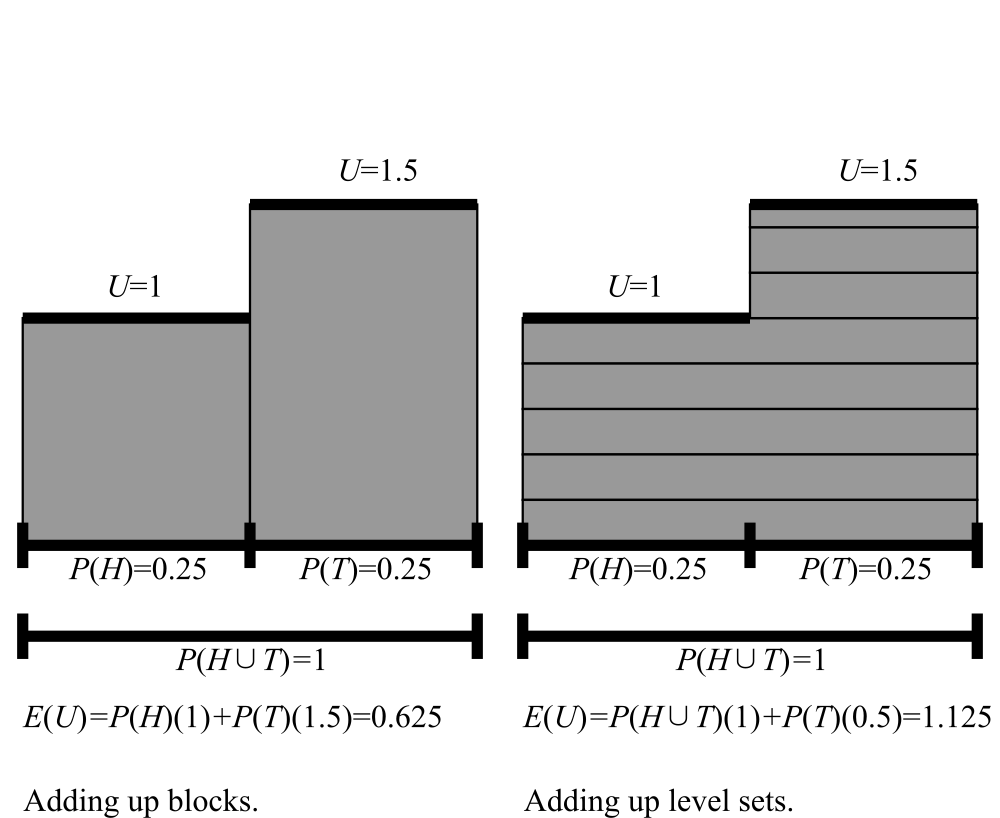## Thursday, November 7, 2019

### Expected utility and inconsistent credences

Suppose that we have a utility function U and an inconsistent credence function P, and for simplicity let’s suppose that our utility function takes on only finitely many values. The standard way of calculating the expected utility of U with respect to P is to look at all the values U can take, multiply each by the credence that it takes that value, and add:

1. E(U)=∑yyP(U = y).

Call this the Block Way or Lebesgue Sums.

Famously, doing this leads to Dutch Books if the credence function fails additivity. But there is another way to calculate the expected utility:

1. E(U)=∫0P(U > y)dy − ∫−∞0P(U < y)dy.

Call this the Level Set Way, because sets of points in a space where some function like U is bigger or smaller than some value are known as level sets.

Here is a picture of the two ways:On the Block Way, we broke up the sample space into chunks where the utility function is constant and calculated the contribution of each chunk using the inconsistent credence function, and then added. On the Level Set Way, we broke it up into narrow strips, and calculated the contribution of each strip, and then added.

It turns out that if the credence function P is at least monotone, so that P(A)≤P(B) if A ⊆ B, a condition strictly weaker than additivity, then an agent who maximizes utilities calculated the Level Set Way will not be Dutch Booked.

Here is another fact about the Level Set Way. Suppose two credence functions U1 and U2 are certain to be close to each other: |U1 − U2|≤ϵ everywhere. Then on the Block Way, their expected utilities may be quite far apart, even assuming monotonicity. On the other hand, on the Level Set Way, their expected utilities are guaranteed to be within ϵ, too. The difference between the two Ways can be quite radical. Suppose a coin is tossed, and the monotone inconsistent credences are:

• tails: 0.01

• neither: 0

Suppose that U1 says that you are paid a constant \$100 no matter what happens. Both the Block Way and the Level Set Way agree that the expected utility is \$100.
But now suppose that U2 says you get paid \$99 on heads and \$101 on tails. Then the Block Way yields:

• E(U2)=0.01 ⋅ 99 + 0.01 ⋅ 101 = 1

while the Level Set Way yields:

• E(U2)=1 ⋅ 99 + 0.01 ⋅ 2 = 99.02

Thus, the Block Way makes the expected value of U2 ridiculously small, and far from that of U1, while the Level Set Way is still wrong—after all, the credences are stupid—but is much closer.

So, it makes sense to think of the Level Set Way as harm reduction for those agents whose credences are inconsistent but still monotone.

That said, many irrational agents will fail monotonicity.

IanS said...

That’s interesting. Note that to apply it, for example, to the roll of a six-sided die, you would need credences not just for the six basic outcomes, but for all 2^6 combinations of them.

Alexander R Pruss said...

On the other hand, the standard procedure means that you need to recalculate whenever you switch to a finer partition of the state space. So, you've done your calculation with one die. But then you find out that you don't know whether it's going to rain tomorrow. So, now, even when the rain is irrelevant to the payoffs, you need to sum utilities over all the atoms, and the atoms are now things of the form (rain/no rain)&(die outcome x). And since your credences may not be additive, the recalculation may change things.

IanS said...

I’m not sure I follow, but I agree with your conclusion. The problem with the Block Way is that is that the calculated expectation can depend on how an event is described. (In your last example \$100 in any case gets expectation \$100, but \$100 on heads, \$100 on tails gets expectation \$2.) It is precisely this feature that permits Dutch books. The Level Set Way blocks Dutch books by defining expectation in a description-independent way.

Here is an interesting point. A standard objection to Dutch book arguments is that you might be prepared to accept each bet of a book individually, but reject the package. The Level Set Way illustrates this as follows.

Take the credences mentioned in your earlier post (0.8 for heads , 0.8 for tails, 1.0 for heads-or-tails, 0.0 for empty). With these credences, using the Level Set Way, you would accept the bet Win \$1 on heads, lose \$2 on tails. You would also accept a similar bet with heads and tails reversed. It seems that the two bets together would make a Dutch book. But they don’t. Using the Level Set Way, if you have already accepted one, you will reject the other. The non-linearity is crucial. Put differently, the vNM independence axiom is violated.

So there is no Dutch book. Still, it does not seem reasonable that you would be prepared to accept either bet.

A general comment: if you know that your credences are inconsistent, wouldn’t you do better to try to reconcile them than to try to calculate with them?

Alexander R Pruss said...

"if you know that your credences are inconsistent, wouldn’t you do better to try to reconcile them than to try to calculate with them?"

Yeah. But what if you don't know whether they are inconsistent?

IanS said...

In my previous comment, I was wrong about the example of credence 0.8 for heads, 0.8 for tails. I was not thinking straight. For what it is worth, what I said would be correct relative to a state in which you had already accepted \$2 on heads and \$2 on tails.

Alexander R Pruss said...

A paper exploring Level Set Integrals for decision theory has just been accepted by Synthese.